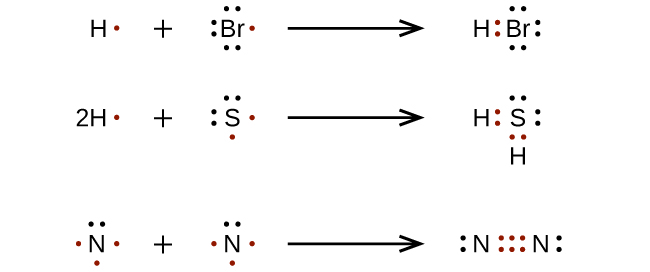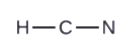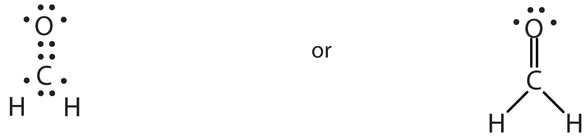# 4.4: Drawing Lewis Structures

Learning Objectives

• To draw Lewis structures.
• To recognize molecules that are likely to have multiple covalent bonds.

#### DRAWING LEWIS STRUCTURES

For very simple molecules and molecular ions, we can write the Lewis structures by merely pairing up the unpaired electrons on the constituent atoms. See these examples:For more complicated molecules and molecular ions, it is helpful to follow the step-by-step procedure outlined here:

1. Determine the total number of valence (outer shell) electrons among all the atoms. For cations, subtract one electron for each positive charge. For anions, add one electron for each negative charge.
2. Draw a skeleton structure of the molecule or ion, arranging the atoms around a central atom. (Generally, the least electronegative element should be placed in the center.) Connect each atom to the central atom with a single bond (one electron pair).
3. Distribute the remaining electrons as lone pairs on the terminal atoms (except hydrogen), completing an octet around each atom.
4. Place all remaining electrons on the central atom.
5. Rearrange the electrons of the outer atoms to make multiple bonds with the central atom in order to obtain octets wherever possible.

Let us determine the Lewis structures of OF2 and HCN as examples in following this procedure:

1. Determine the total number of valence (outer shell) electrons in the molecule or ion. For a molecule, we add the number of valence electrons (use the main group number) on each atom in the molecule. This is the total number of electrons that must be used in the Lewis structure.

O + 2 (F) = OF2 H + C + N = HCN

6e- + (2 x 7e-) = 20e- 1e-+ 4e-+ 5e-= 10e-

2. Draw a skeleton structure of the molecule or ion, arranging the atoms around a central atom and connecting each atom to the central atom with a single (one electron pair) bond. Note that H and F can only form one bond, and are always on the periphery rather than the central atom.3. Distribute the remaining electrons as lone pairs on the terminal atoms (except hydrogen) to complete their valence shells with an octet of electrons.

• In OF2, six electrons are placed on each F.
• In HCN, six electrons placed on N4. Place all remaining electrons on the central atom.

• In OF2, 4 electrons are placed on O.
• In HCN: no electrons remain (the total valence of 10e-is reached) so nothing changes.5. Rearrange the electrons of the outer atoms to make multiple bonds with the central atom in order to obtain octets wherever possible.

• In OF2, each atom has an octet as drawn, so nothing changes.
• In HCN, form two more C–N bondsFinally, check to see if the total number of valence electrons are present in the Lewis structure. And then, inspect if the H atom has 2 electrons surrounding it and if each of the main group atoms is surrounded by 8 electrons.

#### MULTIPLE BONDS

In many molecules, the octet rule would not be satisfied if each pair of bonded atoms shares only two electrons. Review HCN in Step 5 above. Another example is carbon dioxide (CO2). CO2 has a total valence of 4e- + (2 x 6e-) = 16e-. Following steps 1 to 4, we draw the following:This does not give the carbon atom a complete octet; only four electrons are in its valence shell. This arrangement of shared electrons is far from satisfactory.In this case, more than one pair of electrons must be shared between two atoms for both atoms to have an octet. A second electron pair from each oxygen atom must be shared with the central carbon atom shown by the arrows above. A lone pair from each O must be converted into a bonding pair of electrons.In this arrangement, the carbon atom shares four electrons (two pairs) with the oxygen atom on the left and four electrons with the oxygen atom on the right. There are now eight electrons around each atom. Two pairs of electrons shared between two atoms make a double bond between the atoms, which is represented by a double dash:Some molecules contain triple bonds (like HCN, shown above). Triple bonds are covalent bonds in which three pairs of electrons are shared by two atoms. Another compound that has a triple bond is acetylene (C2H2), whose Lewis diagram is as follows:Example $$\PageIndex{1}$$

Draw the Lewis diagram for each molecule.

1. $$\ce{N2}$$
2. $$\ce{CH2O}$$ (The carbon atom is the central atom.) One application of CH2O, also called formaldehyde, is the preservation of biological specimens. Aqueous solutions of CH2O are called formalin and have a sharp, characteristic (pungent) odor.

Solution

1. The total number of electrons is 2 x 5 = 10 electrons. The bond between the two nitrogen atoms is a triple bond. The Lewis diagram for N2is as follows:1. The total number of electrons is 4 x 2(1) + 6 = 12 electrons. In CH2O, the central atom is surrounded by two different types of atoms. The Lewis diagram that fills each atom’s valence electron shell is as follows:Exercise $$\PageIndex{1}$$

Draw the Lewis diagram for each molecule.

1. $$\ce{O2}$$
2. $$\ce{C2H4}$$orororExample $$\PageIndex{2}$$

Which is the correct Lewis structure for N2H2?

A.B.C.Solution

Lewis structure A is the correct answer. It has a total of (2 x 5e-) + (2 x 1e-) = 12e-. Each of the N atoms satisfy the octet requirement and the H atoms follow the duet rule.

Structure B is electron deficient. It has only 10e- instead of 12.

Structure C has 14 (2 extra) electrons. The N atoms do not satisfy the octet.

Exercise $$\PageIndex{2}$$

Which is the correct Lewis structure for NOCl?

A.B.C.Structure A violates the octet rule; N is surrounded by only 6e-.

Structure B violates the octet rule; Cl has 10e- around it. Furthermore, there are a total of 20e- instead of 18e-.

Structure C is the correct structure. It has a total of 6e- + 5e- + 7e- = 18e-. Each atom is surrounded by 8 electrons (octet rule).

## Key Takeaways

• A Lewis structure shows the bonding and nonbonding electrons around individual atoms in a molecule.
• Some molecules must have multiple covalent bonds between atoms to satisfy the octet rule.
• A double bond contains four electrons and a triple bond contains six electrons.

## Exercises

1. What is one clue that a molecule has a multiple bond?

2. Draw the Lewis diagram for each of the following.

a. H2O

b. NH3

c. C2H6

d. CCl4

3. Each molecule contains double bonds. Draw the Lewis diagram for each. The first element is the central atom.

1. CS2
2. C2F4
3. COCl2

4. Each molecule contains multiple bonds. Draw the Lewis diagram for each. Assume that the first element is the central atom, unless otherwise noted.

1. N2
2. CO
3. HCN (The carbon atom is the central atom.)
4. POCl (The phosphorus atom is the central atom.)

5. Explain why hydrogen atoms do not form double bonds.

6. Why is it incorrect to draw a double bond in the Lewis diagram for MgO?

1. If single bonds between all atoms do not give all atoms (except hydrogen) an octet, multiple covalent bonds may be present.
2. a.b.c.d.3. a.b.c.4. a.b.c.d.5. Hydrogen can accept only one more electron; multiple bonds require more than one electron pair to be shared.

6. MgO is an ionic compound (Mg transfers two electrons to O). The electrons are not shared hence it's incorrect to draw a double bond.

This is the Lewis dot structure of MgO.